# Crosscorrelation

In this section we assume you've made your electrophysiological recordings, you've sorted the data into single units and you've constructed individual spike trains and PSTHs, if appropriate. (See the Introduction section above if you don't know what these things are.) Now what?

There's a great deal of interesting stuff to be learned from analyzing simultaneously recorded spike trains. Probably the easiest and most popular analysis involves the construction of the crosscorrelogram. In neurophysiology, the crosscorrelogram is a function which indicates the firing rate of one neuron (the "target" neuron) versus another (the "reference" neuron). Rather than immediately trying to further explain what this means, we'll next describe the manner in which the crosscorrelogram is constructed; it'll be easier to understand what the crosscorrelogram is if you know how to make one.

The crosscorrelogram compares the output of 2 different neurons, so first you need to select the 2 cells you want to analyze. You arbitrarily choose one cell to be the reference cell -- the spikes of this cell's spike train will provide the reference marker. To make the crosscorrelogram, you proceed as follows: line up the 2 spike trains (so that their time markers are vertically aligned); for each spike in the reference spike train, center a window, broken into small segments of time called bins; now examine the target spike train within the window, and increment the bins in which you find any spikes. The procedure is schematized in Figure 5. All it really amounts to is making something like a PSTH for the target neuron, except that instead of a stimulus marker you use the spikes of another neuron as the reference.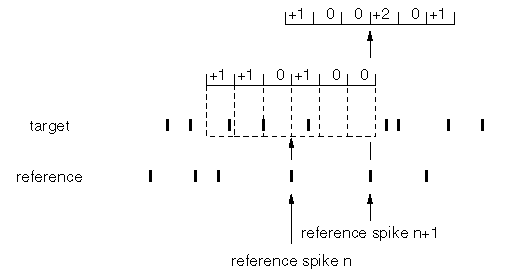Figure 5

The crosscorrelogram thus shows a count of the spikes of the target cell at specific time delays with respect the spikes of the reference cell. The time delay is given by the distance along the horizontal axis, and both "positive" and "negative" delays are recorded, as you can see from Figure 5. Bin counts on the positive side -- that is, counts that occur after time 0, the window center -- mean that the target cell spike came after the reference cell spike; conversely for the negative side. By the way, it may not be completely obvious from the construction method, but if you compute the crosscorrelogram using neuron A as reference and B as the target, and then compute the crosscorrelogram using B as reference and A as the target, you'll get the same thing, only reversed in time (i.e., the horizontal axis).

Recall that PSTHs give some measure of the firing rate or firing probability of a neuron as a function of time, starting with (or around) a reference point, called the stimulus marker. Similarly, crosscorrelograms give some measure of the firing rate or firing probability of the target neuron around the time that the reference neuron fires. Therefore, the crosscorrelogram provides some indication of the dependence, or lack thereof, of the two neurons. If, for instance, the output of neuron A is completely unrelated to neuron B, then (by definition) the spike times of A are just random instants of time from B's point of view; and, if you simply randomly choose times around which to measure a neuron's firing rate, you certainly wouldn't expect that neuron's firing rate to depend on which times you chose. This means that, if you simply pick random times to make the histogram, the firing rate of the neuron should be relatively constant, i.e., the histogram should be flat -- no period of time around the random reference markers should be likely to have more or less spikes than any other periods (within statistical fluctuations). On the other hand, if there is a higher probability that the target cell fires immediately following the reference cell's spike (or marker), then you would see a peak in the crosscorrelogram at that time. See Figure 6. Of course there's also the possibility of troughs in the crosscorrelogram; this is where the target cells' firing rate is decreased with respect to the reference cell's spikes.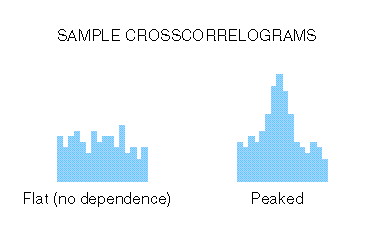Figure 6

It's pretty simple to compute the crosscorrelogram. The problem, though, is how to interpret it. Let's say that you see a peak in the crosscorrelogram for neuron A (target cell) vs. neuron B (reference cell). Is that peak significant, or is it just due to chance? Features of crosscorrelograms, such as peaks or troughs, could be due to a relationship between the spiking activity of the two cells -- which is what you were looking for -- or, alternatively, they could simply arise from a chance arrangement of spikes in the trains, which would not imply an actual relationship between the cells. This is the basic problem of statistical sampling: we've taken a sample of spikes from the two cells and performed a computation (i.e., a statistic) on the data, and we want to know the chance that our result is simply due to random fluctuations (the null hypothesis) or an actual relationship between the 2 neurons (the alternative hypothesis).

Generally, such a statistical test is done when you have presented a stimulus repeatedly for many trials. In this case, you compute the crosscorrelogram over the data segments in which the cells were stimulated. However, there's a bit of a problem here that you must be aware of: when you stimulate the cells that you're recording from, you (generally) elevate their firing rates. If you do this simultaneously in both cells -- which is usually the whole idea of the experiment -- what you are doing is simultaneously increasing the firing rate of both cells at the same time; thus, you've introduced a relationship between the firing probabilities of the cells, just by co-stimulating them. This will cause a peak in the crosscorrelogram, all by itself. But this is most certainly not the sort of relationship you are looking for. In neural physiology, firing rate relationships between cells are taken to be evidence of either synaptic connectivity between the cells or common input (see below); you don't care to know about relationships that the experimenter artificially introduced in the course of the experiment!

Therefore, the covariation in firing rates of the two stimulated cells must be removed before considering the peak to be relevant. The easiest way to "correct" for this stimulus-induced relationship is to use the shift predictor. What the shift predictor theoretically tells you is the shape of the crosscorrelogram based solely on firing rates of the cells, in the absence of any physical relationship (in the form of synaptic connectivity or common input) between the cells. The shift predictor is constructed by taking the two spike trains and shifting one of them a certain distance in time, so that the spike train of one trial of one neuron corresponds with a different trial of the other spike train. Figure 7 shows a shift of exactly 1 trial. The crosscorrelogram is recomputed using the shifted spike train pair, and this correlogram is subtracted from the original crosscorrelogram, bin by bin.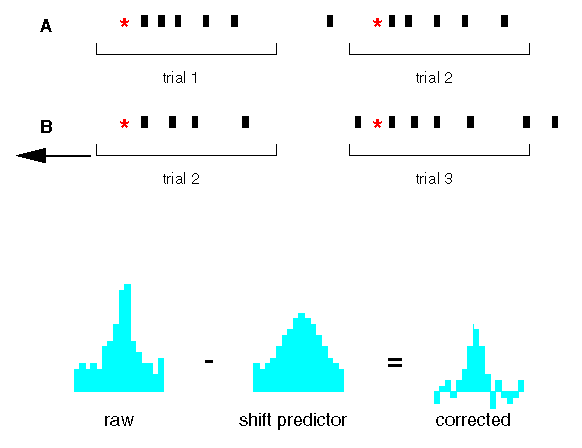Figure 7

So how does this remove the peak in the original correlogram that was due to co-stimulation of the cells? The average and stimulus-modulated firing rates of the cells were not altered by the shifting process; what was destroyed was any physiological relationship between the individual spikes of the neurons. This is because synaptic connectivity or common input occur on time scales that are generally very small compared to the interstimulus times, and it's therefore highly unlikely that spikes from one stimulus trial are affected by the previous or following trial's spike trains. The shift predictor is therefore a measure of coincidences caused by covariation of the firing rates by action of the stimulus. Note that the shift predictor does not measure sources of covariation in the spike trains that are not time-locked to the stimulus. (You should also be aware that, due to noise, etc., the shift predictor is generally computed by averaging over all possible trial shifts, not just one. Also, for computational efficiency, the calculation is generally done by taking the cross product of the two cells' PSTHs, which amounts to the same thing.)

It's also important to understand that the procedures described in the paragraph above may cause trouble if the data are nonstationary. (See below.)

Okay, so now our crosscorrelogram should contain only contributions from physiological mechanisms not directly related to the stimulus. But the peak or trough in the corrected correlogram could still be due to chance correlations in the spike trains (assuming there is a peak or trough -- in many cases, you unfortunately see nada). So now comes the statistical test.

We're going to assume that the counts in the crosscorrelogram bins are Poisson processes (and that's generally a good assumption); therefore, you can use the Poisson distribution to test the significance of the corrected crosscorrelogram bin counts. You perform this test on each bin. Let's say, for instance, that a particular bin in the corrected crosscorrelogram has 8 counts and that the shift predictor for the corresponding bin has 20 counts. (Meaning, of course, the original crosscorrelogram bin has 28 counts.) The null hypothesis assumes that the spike trains are independent, with only stimulus-induced firing rate correlations; if this is true, we would expect the original crosscorrelogram bin count to have the value indicated by the shift predictor, with some degree of statistical fluctuations. In other words, our null hypothesis for this bin is that the bin count distribution has a mean of 20 and a standard deviation (given the fact that we've assumed a Poisson distribution) equal to the square root of 20. It is easy to compute the chance of getting a bin count of 28 under such circumstances. If the chance is quite low, say, below p = 0.01, we reject the null hypothesis, indicating that this bin has a count that is not as expected for 2 independent spike trains.

The statistical test is then performed on each bin, always assuming a Poisson distribution of counts with a mean based on the shift predictor for that bin. Unfortunately, the question of the dependent or independent nature of the spike trains is not clear from this bin by bin test; you would not, for instance, claim that the spike trains are dependent simply because the null hypothesis was rejected in only one bin test. After all, if you accept significance based on p = 0.01, then on average you expect to be wrong 1 out of 100 times; so if your window includes 100 bins, you're probably going to get at least one false positive! Essentially what you're looking for is a few consecutive bins which have such unusually extreme values (as judged by the statistical test) that you can safely reject the hypothesis that the two spike trains are completely independent.

Now that you're reasonably sure that the peak (or trough) in the crosscorrelogram is meaningful, what does it mean? Physiologically, there are 2 basic ways that neural spike trains can become correlated: neurons can have a synaptic connection, or they can have common input. Both of these are important physiological relationships to know, but synaptic coupling is probably the most interesting. Synaptic coupling just means that one cell makes a synapse with the other. Common input simply means that both cells receive synaptic input from one or more different cells. Figure 8 shows these relationships schematically.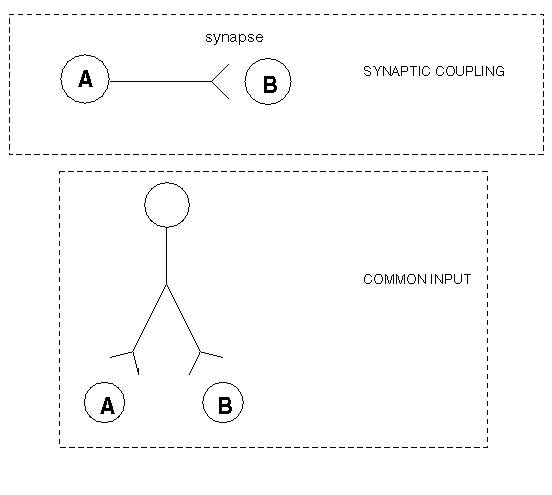Figure 8

Given these 2 possibilities, how can you tell which is which from the crosscorrelgram peak or trough? Unfortunately, you really can't be sure; conventional wisdom, however, suggests that a sharp peak is due to synaptic coupling, whereas a broad peak is probably due to common input. This is because common input -- coming, in all likelihood, from many cells -- is probably temporally spread out over time. Another interesting thing to look for is if the peak is centered or not in the crosscorrelogram, i.e., if the peak occurs at some delay time other than zero. If the peak is noncentered then this may suggest synaptic coupling, where the delay is due to the axonal conduction as well as the delay due to the synapse. However, since common inputs to the cells can be differentially delayed, you can't be sure that an offcenter peak is due to synaptic coupling.

Discovering relationships between neurons is important, since behavior results not from a single cell but from collections, or networks, of neurons acting together. From correlation analysis we can get some idea of how specific neurons interact during the process of analyzing sensory information or producing a complex goal-directed movement. We would also anticipate that these interactions will change depending on what the networks are doing; thus, the particular computations that a neural network is doing may be better understood by noting the particular way that neurons are interacting, as revealed by correlation analysis.

Unfortunately, the simple crosscorrelogram is limited in the amount of information it can provide. You might like to know, for example, whether the relationship between the spikes of the 2 cells was changing over the time course of the stimulus response; the crosscorrelogram gives no clue here. Another limitation of the crosscorrelogram is that you can compare only 2 cells at a time. Therefore, other tools for analyzing spike trains have been developed, as descibed below. First, however, we must give the obligatory caveats of crosscorrelation analysis; like all tools, it's not useful in all situations, and it can be easily abused.

## Caveats

### Nonstationarities

When discussing the crosscorrelation analysis of neural data, we didn't make any explicit assumptions about the structure of the spike trains. But we were tacitly making a very important assumption about the data when we used the statistical tests to determine significance of the results -- we assumed that the statistics of the spike trains were not changing over time. (More technically: we were assuming weak stationarity, which means that the first and second order statistics were constant over the course of the experiment.)

This paragraph contains a brief digression on what the term "statistics" means when applied to spike trains; it can be safely skipped for those people with low curiosity coefficients. Spike trains can be thought of as random processes; in many cases, in fact, neural spike trains can be realistically modeled by randomly pulling interspike intervals from an exponential distribution (which implies a Poisson process for the production of the spikes). Random processes have statistical properties, like the mean, the variance, etc. (more technically: they have moments). The defining characteristic of a random process is that it can produce many different sequences of events (the different sequences are called realizations), and which sequence it produces is not entirely predictable in advance; thus the random process is only probabilistic in its output. Any one realization of a random process (a particular spike train, for instance) may deviate from the statistical properties of the process, since the properties only describe the ensemble of realizations. (The ensemble refers to all possible realizations that can be produced by the process). In neurophysiological terms, we identify the random process as the neuron: a neuron is capable of producing a wide range of different spike trains (the ensemble), even in response to identical stimuli; and these spike trains, taken together and averaged, have certain properties which we think of as belonging to the neuron in question (or rather, to the neuron's spike train generation mechanisms, which is the actual random process in which we've recorded a few spike train realizations).

So our assumption is that these statistics don't change much over the course of the experiment, i.e., the process is "stationary". This amounts to the assumption that the neural properties are fairly static, at least with respect to experimental time scales. As you might imagine, however, this can be rather difficult to detect, since individual spike trains are generally quite variable.

In our discussion above, we've already run into what, strictly speaking, amounts to a violation of that assumption. One very basic spike train statistic is firing rate, and when we stimulate a neuron, our general intent is to change the firing rate. We basically deal with this "nonstationarity" by segmenting the data, as we did above, into periods of time around each stimulus repetition, and analyzing these segments. We then assumed that the neuron's properties were repeatedly varying only in a way that is time-locked to the stimulus.

Minor violations of stationarity probably aren't very serious. But for major nonstationarities, the result can be catastrophic. Consider the ultimate nonstationarity: during the course of the experiment, the neurons die. (That ought to change their statistical properties!) Let's say we used 100 trials in that experiment, and that on the 50th trial, the neurons go away. So our experiment was just fine for 50 trials, but yielded nada for the last 50. What happens when we compute the average shift predictor? Well, it boils down to obtaining an "average" by dividing the sum by 100 instead of 50 -- as you might expect, that "average" is going to be a bit too small. When you subtract this tiny shift predictor from the raw crosscorrelogram, a peak always remains. Quite a peak -- very broad! (See Figure 9.)Figure 9

The nonstationarity generated by the deaths of both neurons is a bit extreme, of course. (And was picked as an example because of the computational simplicity of the results.) Other, less severe nonstationarities -- particularly those that don't influence both spike trains in the same manner, unlike the example above -- are much less predictable in the nature of their effects on the data analysis. More realistic sources of nonstationarities are slow changes in the operating "state" of the nervous system; for example, modulations by slow-acting neurotransmitters like dopamine or serotonin. The subject of nonstationarities, particularly their effects as well as their detection and subsequent correction, is terra incognita at the moment, and awaiting future exploration!

Going back to our (contrived) example, note that the bin counts of the "corrected" crosscorrelogram in Figure 9 do not go to zero at the ends of the window. Bins far removed from the zero coincidence should be close to zero in a truly corrected crosscorrelogram. This is because, as we have stated earlier, physiological mechanisms which produce relationships in spike trains do not act over long periods of time; thus, at large intervals, spikes of 2 neurons should not be correlated. Hence, at large delays, the crosscorrelogram should merely be influenced by the average firing rate of the cells, and this is exactly what should be subtracted by the shift predictor.

Note: It's perfectly normal for raw crosscorrelograms to be nonzero at the ends of the window. Some researchers don't subtract the shift predictor if it's relatively flat (which would, in effect, be subtracting a constant from the raw crosscorrelogram) and thus these researchers report raw correlograms, which don't fall to zero at long delays.

So how do you detect nonstationarities? As mentioned above, that can be a tough problem, and one that, in general, we don't really know how to solve. But you can certainly detect obvious violations of stationarity -- one simple way is just to print a rasterplot of the spiketrains, with each stimulus trial lined up on the page. Eyeballing the result, you should not see any gaps containing only white space; there should be a relatively smooth distribution of spikes.

Another interesting problem is what you do when there are nonstationarities in the data. That -- as you might anticipate -- is another tough problem. Our lab, among others, are currently working on some possible solutions.

### The Case of the Drooping Crosscorrelogram

What happens if you make the crosscorrelogram window large with respect to the stimulus time interval? (See Figure 10.) In this case, you tend to get a "drooping" crosscorrelogram, since the spikes at the beginning and ending of the stimulus time interval are surrounding by stimulus-elevated firing rates only on one side. The result is often a broad peak in the crosscorrelogram, which can unfortunately be occasionally mistaken for a physiological relationship.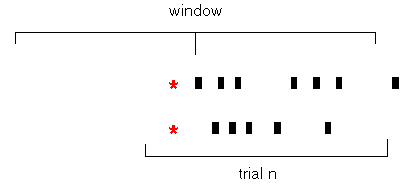Figure 10

### Small Sample Size

You would, of course, prefer to have infinite amounts of data from which to draw your inferences. In actual practice, however, you fall a bit short of that mark. But one should be very careful how one interprets results coming from a small sample. How small is too small? There really isn't a very quantitative answer to this question. One rule of thumb: the firing rate should be a relatively smooth function of time, and if your PSTH -- which is, of course, an estimate of the cell's firing rate -- is quite hairy, with some bins sticking up and others very depressed, some degree of concern is justified. (Of course, hairy PSTHs could be caused by oscillatory activity as well, as long as it's time locked to the stimulus marker.) In fact, some people smooth the PSTHs they report -- in its simplest form, what this amounts to is replacing each bin by an average of the surrounding bins, thus averaging out local fluctuations.

Kyle Kirkland

Back Home